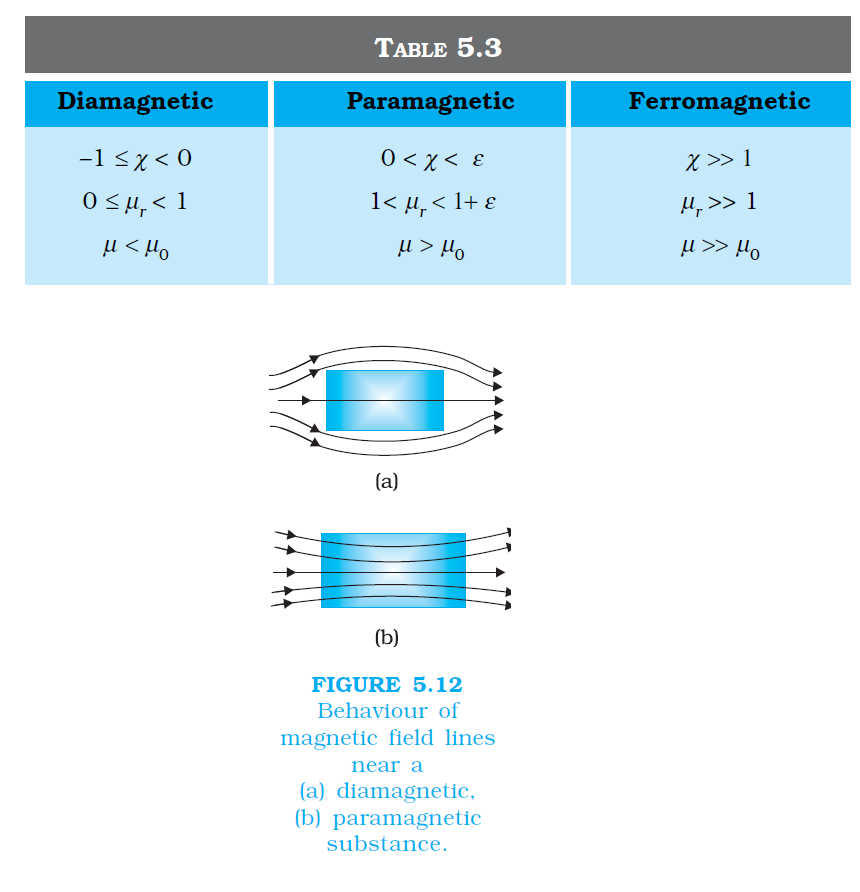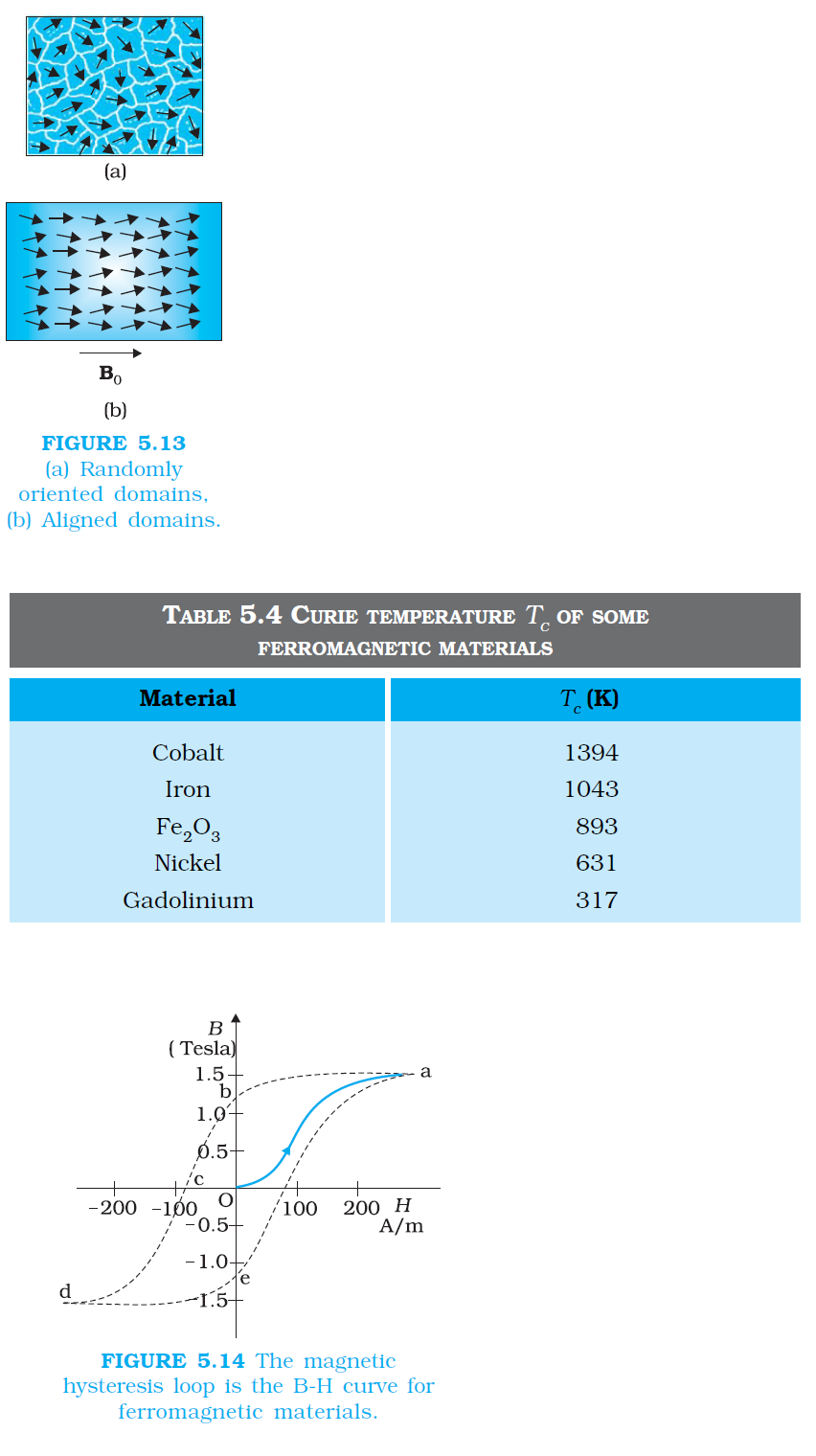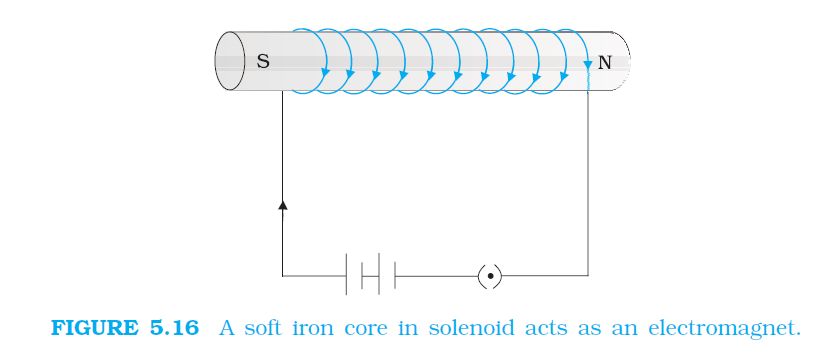Physics MAGNETISATION AND MAGNETIC INTENSITY,MAGNETISATION AND MAGNETIC INTENSITY, FERROMAGNETISM, PERMANENT MAGNETS AND ELECTROMAGNETS FOR CBSE-NCERT 3

### Topic covered

color{blue}{star} MAGNETISATION AND MAGNETIC INTENSITY
color{blue}{star} MAGNETISATION AND MAGNETIC INTENSITY
color{blue}{star} FERROMAGNETISM
color{blue}{star} PERMANENT MAGNETS AND ELECTROMAGNETS

### MAGNETISATION AND MAGNETIC INTENSITYcolor{blue} ✍️ The earth abounds with a bewildering variety of elements and compounds. In addition, we have been synthesising new alloys, compounds and even elements.

color {blue}{➢➢} One would like to classify the magnetic properties of these substances. In the present section, we define and explain certain terms which will help us to carry out this exercise.

color{blue} ✍️ We have seen that a circulating electron in an atom has a magnetic moment. In a bulk material, these moments add up vectorially and they can give a net magnetic moment which is non-zero. We define magnetisation color{blue}(M) of a sample to be equal to its net magnetic moment per unit volume:

color{blue}(M = (m_(n e t))/V)

.................(5.11)

color {blue}{➢➢} color{blue}(M) is a vector with dimensions color{blue}(L^(-1) A) and is measured in a units of color{blue}(A m^(–1)).

color {blue}{➢➢} Consider a long solenoid of color{blue}(n) turns per unit length and carrying a current color{blue}(I). The magnetic field in the interior of the solenoid was shown to be given by

color{blue}(B_0 = mu_0 nI)

.............(5.12)

color {blue}{➢➢} If the interior of the solenoid is filled with a material with non-zero magnetisation, the field inside the solenoid will be greater than color{blue}(B_0). The net color{blue}(B) field in the interior of the solenoid may be expressed as

color{blue}(B = B_0 + B_m)

...........(5.13)

color {blue}{➢➢} where color{blue}(B_m) is the field contributed by the material core. It turns out that this additional field color{blue}(B_m) is proportional to the magnetisation color{blue}(M) of the material and is expressed as

color{blue}(B_m = μ_0 M)

..........(5.14)

color {blue}{➢➢} where color{blue}(μ_0) is the same constant (permeability of vacuum) that appears in
Biot-Savart’s law. It is convenient to introduce another vector field color{blue}(H), called the magnetic intensity, which is defined by

color{blue}(H= B/(mu_0) -M)

..............(5.15)

color {blue}{➢➢} where color{blue}(H) has the same dimensions as color{blue}(M) and is measured in units of color{blue}(A m^(–1)). Thus, the total magnetic field color{blue}(B) is written as

color{blue}(B = mu_0(H+M))

............(5.16)

color {blue}{➢➢} We repeat our defining procedure. We have partitioned the contribution to the total magnetic field inside the sample into two parts: one, due to external factors such as the current in the solenoid. This is represented by color{blue}(H).

color {blue}{➢➢} The other is due to the specific nature of the magnetic material, namely color{blue}(M). The latter quantity can be influenced by external factors. This influence is mathematically expressed as

color{blue}(M = χ H)

.....................(5.17)

color {blue}{➢➢} where color{blue}(χ) , a dimensionless quantity, is appropriately called the magnetic susceptibility. It is a measure of how a magnetic material responds to an external field. Table 5.2 lists color{blue}(χ) for some elements. It is small and positive for materials, which are called paramagnetic.

color {blue}{➢➢}It is small and negative for materials, which are termed diamagnetic. In the latter case color{blue}(M) and color{blue}(H) are opposite in direction. From Eqs. (5.16) and (5.17) we obtain,

color{blue}(B=mu_0 (1+χ)H)

...................(5.18)

color{blue}(= mu_0mu_rH)

color{blue}(= muH)

....................(5.19)

color {blue}{➢➢}where color{blue}(μ_r= 1 + χ,) is a dimensionless quantity called the relative magnetic permeability of the substance. It is the analog of the dielectric constant in electrostatics. The magnetic permeability of the substance is color{blue}(μ) and it has the same dimensions and units as color{blue}(μ_0).

color{blue}(mu=mu_0 mu_r = mu_0 (1+χ))

color {blue}{➢➢}The three quantities color{blue}(χ, μ_r) and color{blue}(μ) are interrelated and only one of them is independent. Given one, the other two may be easily determined.Q 3114291150A solenoid has a core of a material with relative permeability 400. The windings of the solenoid are insulated from the core and carry a current of 2A. If the number of turns is 1000 per metre, calculate (a) H, (b) M, (c) B and (d) the magnetising current I_m.
Class 12 Chapter example 10Solution:

(a) The field H is dependent of the material of the core, and is = nI = 1000 × 2.0 = 2 ×10^3 A//m.
(b) The magnetic field B is given by B = μr μ0 H
= 400 × 4π ×10–7 (N//A^2) × 2 × 103 (A//m)
= 1.0 T
(c) Magnetisation is given by
M = (B– μ0 H)// μ0
= (μr μ0 H–μ0 H)/μ0 = (μr – 1)H = 399 × H
≅ 8 × 105 A//m
(d) The magnetising current I_M is the additional current that needs
to be passed through the windings of the solenoid in the absence
of the core which would give a B value as in the presence of the
core. Thus B = μr n0 (I + I_M). "Using" I = 2A, B = 1 T, "we get" I_M = 794 A.

### MAGNETIC PROPERTIES OF MATERIALScolor{blue} ✍️The discussion in the previous section helps us to classify materials as diamagnetic, paramagnetic or ferromagnetic. In terms of the susceptibility color{blue}(χ) , a material is diamagnetic if color{blue}(χ) is negative, para- if color{blue}(χ) is positive and small, and ferro- if color{blue}(χ) is large and positive.

color {blue}{➢➢}A glance at Table 5.3 gives one a better feeling for these materials. Here ε is a small positive number introduced to quantify paramagnetic materials. Next, we describe these materials in some detail.color{green}bbul("Diamagnetism")
color {blue}{➢➢}Diamagnetic substances are those which have tendency to move from stronger to the weaker part of the external magnetic field. In other words, unlike the way a magnet attracts metals like iron, it would repel a diamagnetic substance.

color {blue}{➢➢}Figure 5.12(a) shows a bar of diamagnetic material placed in an external magnetic field. The field lines are repelled or expelled and the field inside the material is reduced. In most cases, as is evident from Table 5.2, this reduction is slight, being one part in color{blue}(10^5). When placed in a non-uniform magnetic field, the bar will tend to move from high to low field.color {blue}{➢➢}The simplest explanation for diamagnetism is as follows. Electrons in an atom orbiting around nucleus possess orbital angular momentum. These orbiting electrons are equivalent to current-carrying loop and thus possess orbital magnetic moment. Diamagnetic substances are the ones in which resultant magnetic moment in an atom is zero.

color {blue}{➢➢}When magnetic field is applied, those electrons having orbital magnetic moment in the same direction slow down and those in the opposite direction speed up. This happens due to induced current in accordance with Lenz’s law which you will study in Chapter 6.

color {blue}{➢➢}Thus, the substance develops a net magnetic moment in direction opposite to that of the applied field and hence repulsion.

color {blue}{➢➢}Some diamagnetic materials are bismuth, copper, lead, silicon, nitrogen (at STP), water and sodium chloride. Diamagnetism is present in all the substances. However, the effect is so weak in most cases that it gets shifted by other effects like paramagnetism, ferromagnetism, etc.

color {blue}{➢➢}The most exotic diamagnetic materials are superconductors. These are metals, cooled to very low temperatures which exhibits both perfect conductivity and perfect diamagnetism.

color {blue}{➢➢} Here the field lines are completely expelled! color{blue}(χ = –1) and color{blue}(μ_r = 0) A superconductor repels a magnet and (by Newton’s third law) is repelled by the magnet. The phenomenon of perfect diamagnetism in superconductors is called the Meissner effect, after the name of its discoverer.

color {blue}{➢➢}Superconducting magnets can be gainfully exploited in variety of situations, for example, for running magnetically levitated superfast trains. 5.6.2 Paramagnetism

color{green}bbul("Paramagnetism")
color {blue}{➢➢}Paramagnetic substances are those which get weakly magnetised when placed in an external magnetic field. They have tendency to move from a region of weak magnetic field to strong magnetic field, i.e., they get weakly attracted to a magnet.

color {blue}{➢➢}The individual atoms (or ions or molecules) of a paramagnetic material possess a permanent magnetic dipole moment of their own. On account of the ceaseless random thermal motion of the atoms, no net magnetisation is seen.

color{blue} ✍️In the presence of an external field color{blue}(B_0), which is strong enough, and at low temperatures, the individual atomic dipole moment can be made to align and point in the same direction as color{blue}(B_0). Figure 5.12(b) shows a bar of paramagnetic material placed in an external field.

color {blue}{➢➢}The field lines gets concentrated inside the material, and the field inside is enhanced. In most cases, as is evident from Table 5.2, this enhancement is slight, being one part in color{blue}(10^5).

color {blue}{➢➢}When placed in a non-uniform magnetic field, the bar will tend to move from weak field to strong. Some paramagnetic materials are aluminium, sodium, calcium, oxygen (at STP) and copper chloride. Experimentally, one finds that the magnetisation of a paramagnetic material is inversely proportional to the absolute temperature color{blue}(T),

color{blue}(M = C(B_0)/T)

......................[5.20 (a)]

color {blue}{➢➢}or equivalently, using Eqs. (5.12) and (5.17)

color{blue}(χ= C(mu_0)/T)

.......................... [5.20 (b)]

color {blue}{➢➢}This is known as Curie’s law, after its discoverer Pieree Curie (1859- 1906). The constant color{blue}(C) is called Curie’s constant. Thus, for a paramagnetic material both color{blue}(χ) and color{blue}(μ_r) depend not only on the material, but also (in a simple fashion) on the sample temperature. As the field is increased or the temperature is lowered, the magnetisation increases until it reaches the saturation value Ms, at which point all the dipoles are perfectly aligned with the field. Beyond this, Curie’s law [Eq. (5.20)] is no longer valid.

### Ferromagnetismcolor{blue} ✍️ Ferromagnetic substances are those which gets strongly magnetised when placed in an external magnetic field. They have strong tendency to move from a region of weak magnetic field to strong magnetic field, i.e., they get strongly attracted to a magnet.

color {blue}{➢➢}The individual atoms (or ions or molecules) in a ferromagnetic material possess a dipole moment as in a paramagnetic material. However, they interact with one another in such a way that they spontaneously align themselves in a common direction over a macroscopic volume called domain.

color{blue} ✍️The explanation of this cooperative effect requires quantum mechanics and is beyond the scope of this textbook. Each domain has a net magnetisation. Typical domain size is 1mm and the domain contains about color{blue}(10^11) atoms. In the first instant, the magnetisation varies randomly from domain to domain and there is no bulk magnetisation.

color {blue}{➢➢} This is shown in Fig. 5.13(a). When we apply an external magnetic field color{blue}(B_0), the domains orient themselves in the direction of color{blue}(B_0) and simultaneously the domain oriented in the direction of color{blue}(B_0) grow in size.

color {blue}{➢➢}This existence of domains and their motion in color{blue}(B_0) are not speculations. One may observe this under a microscope after sprinkling a liquid suspension of powdered ferromagnetic substance of samples. This motion of suspension can be observed. Figure 5.12(b) shows the situation when the domains have aligned and amalgamated to form a single ‘giant’ domain.color {blue}{➢➢}Thus, in a ferromagnetic material the field lines are highly concentrated. In non-uniform magnetic field, the sample tends to move towards the region of high field. We may wonder as to what happens when the external field is removed.

color{blue} ✍️In some ferromagnetic materials the magnetisation persists. Such materials are called hard magnetic materials or hard ferromagnets. Alnico, an alloy of iron, aluminium, nickel, cobalt and copper, is one such material.

color {blue}{➢➢}The naturally occurring lodestone is another. Such materials form permanent magnets to be used among other things as a compass needle. On the other hand, there is a class of ferromagnetic materials in which the magnetisation disappears on removal of the external field.

color{blue} ✍️Soft iron is one such material. Appropriately enough, such materials are called soft ferromagnetic materials. There are a number of elements, which are ferromagnetic: iron, cobalt, nickel, gadolinium, etc. The relative magnetic permeability is >1000!

color {blue}{➢➢}The ferromagnetic property depends on temperature. At high enough temperature, a ferromagnet becomes a paramagnet. The domain structure disintegrates with temperature.

color {blue}{➢➢}This disappearance of magnetisation with temperature is gradual. It is a phase transition reminding us of the melting of a solid crystal. The temperature of transition from ferromagnetic to paramagnetism is called the Curie temperature color{blue}(T_c).

color {blue}{➢➢}Table 5.4 lists the Curie temperature of certain ferromagnets. The susceptibility above the Curie temperature, i.e., in the paramagnetic phase is described by,

color{blue}(χ= C/(T-T_C) (T > T_C))

....................(5.21)color{blue} ✍️The relation between color{blue}(B) and color{blue}(H) in ferromagnetic materials is complex. It is often not linear and it depends on the magnetic history of the sample. Figure 5.14 depicts the behaviour of the material as we take it through one cycle of magnetisation.color{blue} ✍️ Let the material be unmagnetised initially. We place it in a solenoid and increase the current through the solenoid.

color {blue}{➢➢} The magnetic field color{blue}(B) in the material rises and saturates as depicted in the curve color{blue}(Oa). This behaviour represents the alignment and merger of domains until no further enhancement is possible.

color {blue}{➢➢} It is pointless to increase the current (and hence the magnetic intensity color{blue}(H) ) beyond this.

color {blue}{➢➢}Next, we decrease color{blue}(H) and reduce it to zero. At color{blue}(H = 0, B ≠ 0). This is represented by the curve color{blue}(ab). The value of color{blue}(B) at color{blue}(H = 0) is called retentivity or remanence. In Fig. 5.14, color{blue}(B_R ~ 1.2 T), where the subscript color{blue}(R) denotes retentivity. The domains are not completely randomised even though the external driving field has been removed.

color {blue}{➢➢} Next, the current in the solenoid is reversed and slowly increased. Certain domains are flipped until the net field inside stands nullified. This is represented by the curve color{blue}(bc).

color {blue}{➢➢}The value of color{blue}(H) at color{blue}(c) is called coercivity. In Fig. 5.14 color{blue}(H_c ~ –90 A m^(–1)). As the reversed current is increased in magnitude, we once again obtain saturation. The curve color{blue}(cd) depicts this. The saturated magnetic field color{blue}(B_s ~ 1.5 T).

color {blue}{➢➢}Next, the current is reduced (curve color{blue}(de)) and reversed (curve color{blue}(ea)). The cycle repeats itself. Note that the curve color{blue}(Oa) does not retrace itself as color{blue}(H) is reduced. For a given value of color{blue}(H, B) is not unique but depends on previous history of the sample. This phenomenon is called hysterisis. The word hysterisis means lagging behind (and not "history").

Q 3174291156A domain in ferromagnetic iron is in the form of a cube of side length 1μm. Estimate the number of iron atoms in the domain and the maximum possible dipole moment and magnetisation of the domain. The molecular mass of iron is 55 g/mole and its density is 7.9 g//cm^3. Assume that each iron atom has a dipole moment of 9.27×10^(–24) A m^2.
Class 12 Chapter example 11Solution:

The volume of the cubic domain is

V = (10^(–6) m)^3 = 10^(–18) m3 = 10^(–12) cm^3
Its mass is volume × density = 7.9 g cm^(–3) × 10^(–12) cm^3= 7.9 × 10^(–12) g
It is given that Avagadro number (6.023 × 10^23) of iron atoms have a mass of 55 g. Hence, the number of atoms in the domain is

N = (7.8 xx10^(-12)xx6.023xx10^(23))/(55)

= 8.65 × 10^10 atoms
The maximum possible dipole moment mmax is achieved for the
(unrealistic) case when all the atomic moments are perfectly aligned.
Thus,

m_(max) = (8.65 × 10^10) × (9.27 × 10^(–24))
= 8.0 × 10^(–13) A m^2
The consequent magnetisation is

M_max = m_max/Domain volume
= 8.0 × 10^(–13) Am^2//10^(–18) m^3
= 8.0 × 105 Am^(–1)

### PERMANENT MAGNETS AND ELECTROMAGNETScolor{blue} ✍️ Substances which at room temperature retain their ferromagnetic property for a long period of time are called permanent magnets.

color {blue}{➢➢}Permanent magnets can be made in a variety of ways. One can hold an iron rod in the north-south direction and hammer it repeatedly. The method is illustrated in Fig. 5.15. The illustration is from a 400 year old book to emphasise that the making of permanent magnets is an old art.

color {blue}{➢➢}One can also hold a steel rod and stroke it with one end of a bar magnet a large number of times, always in the same sense to make a permanent magnet.color {blue}{➢➢}An efficient way to make a permanent magnet is to place a ferromagnetic rod in a solenoid and pass a current. The magnetic field of the solenoid magnetises the rod.

color {blue}{➢➢}The hysteresis curve (Fig. 5.14) allows us to select suitable materials for permanent magnets. The material should have high retentivity so that the magnet is strong and high coercivity so that the magnetisation is not erased by stray magnetic fields, temperature fluctuations or minor mechanical damage.

color {blue}{➢➢}Further, the material should have a high permeability. Steel is one-favoured choice. It has a slightly smaller retentivity than soft iron but this is outweighed by the much smaller coercivity of soft iron. Other suitable materials for permanent magnets are alnico, cobalt steel and ticonal.

color {blue}{➢➢}Core of electromagnets are made of ferromagnetic materials which have high permeability and low retentivity. Soft iron is a suitable material for electromagnets. On placing a soft iron rod in a solenoid and passing a current, we increase the magnetism of the solenoid by a thousand fold.

color {blue}{➢➢}When we switch off the solenoid current, the magnetism is effectively switched off since the soft iron core has a low retentivity. The arrangement is shown in Fig. 5.16.color{blue} ✍️ In certain applications, the material goes through an ac cycle of magnetisation for a long period. This is the case in transformer cores and telephone diaphragms.

color {blue}{➢➢}The hysteresis curve of such materials must be narrow. The energy dissipated and the heating will consequently be small. The material must have a high resistivity to lower eddy current losses. You will study about eddy currents in Chapter 6.

color {blue}{➢➢}Electromagnets are used in electric bells, loudspeakers and telephone diaphragms. Giant electromagnets are used in cranes to lift machinery, and bulk quantities of iron and steel.